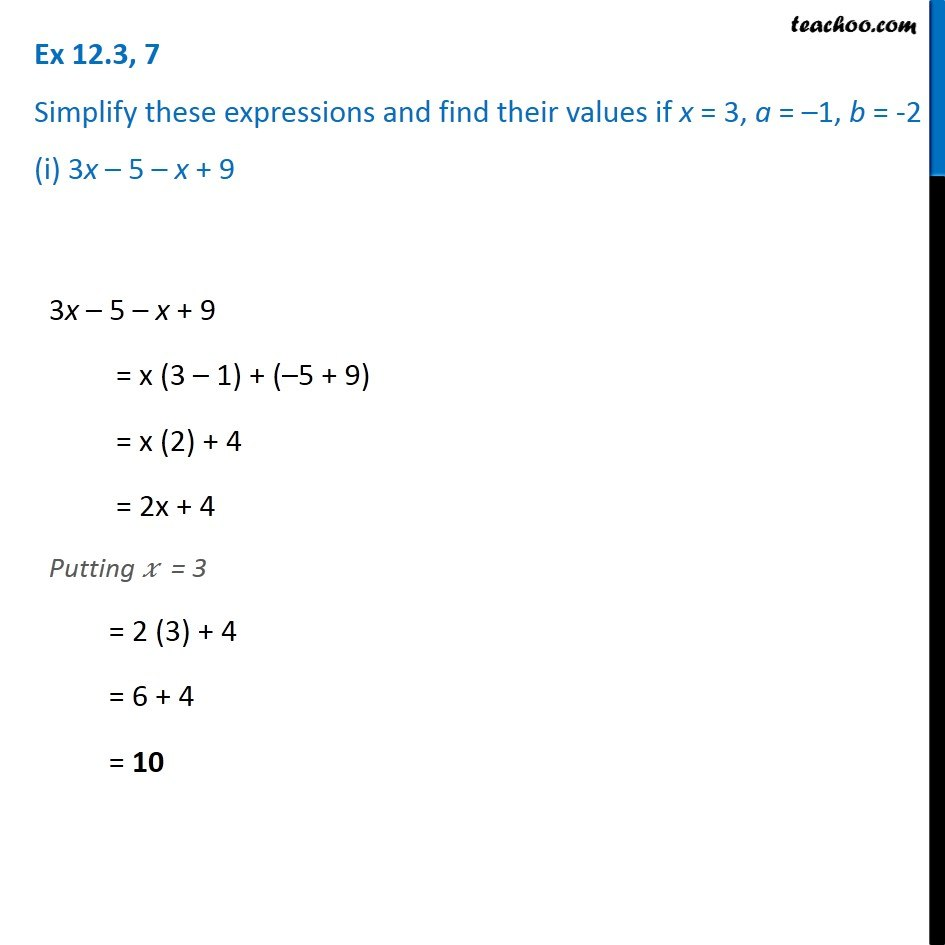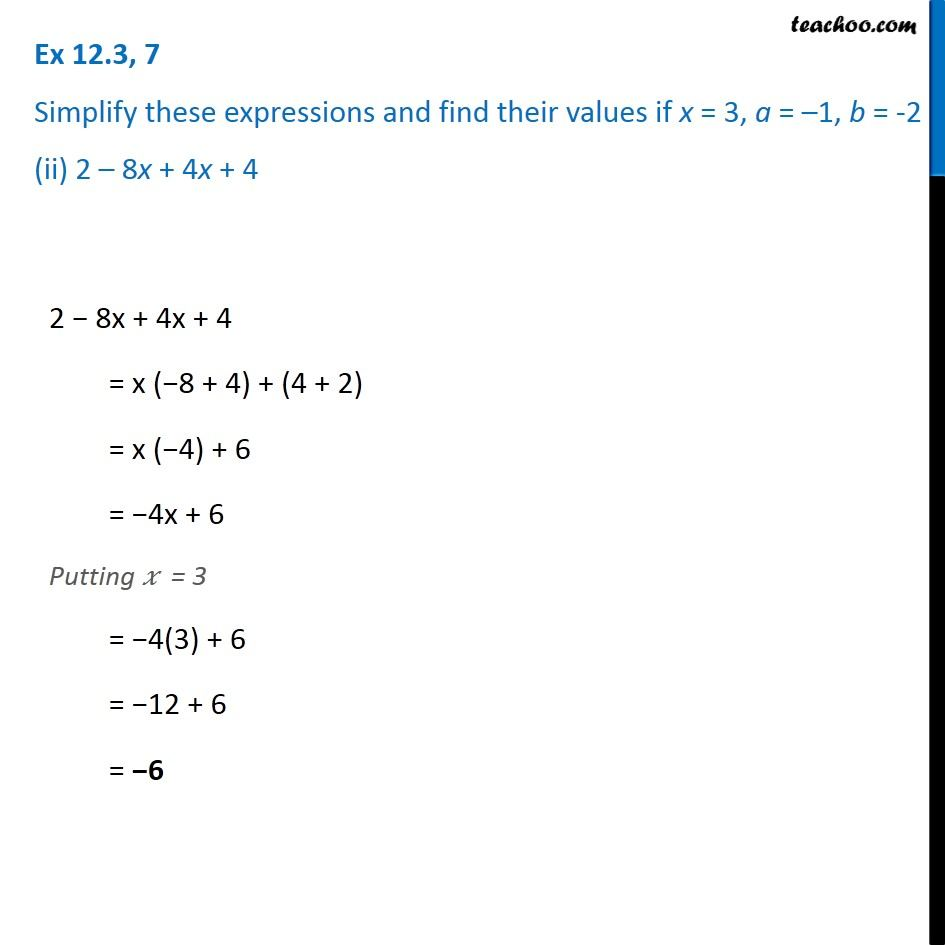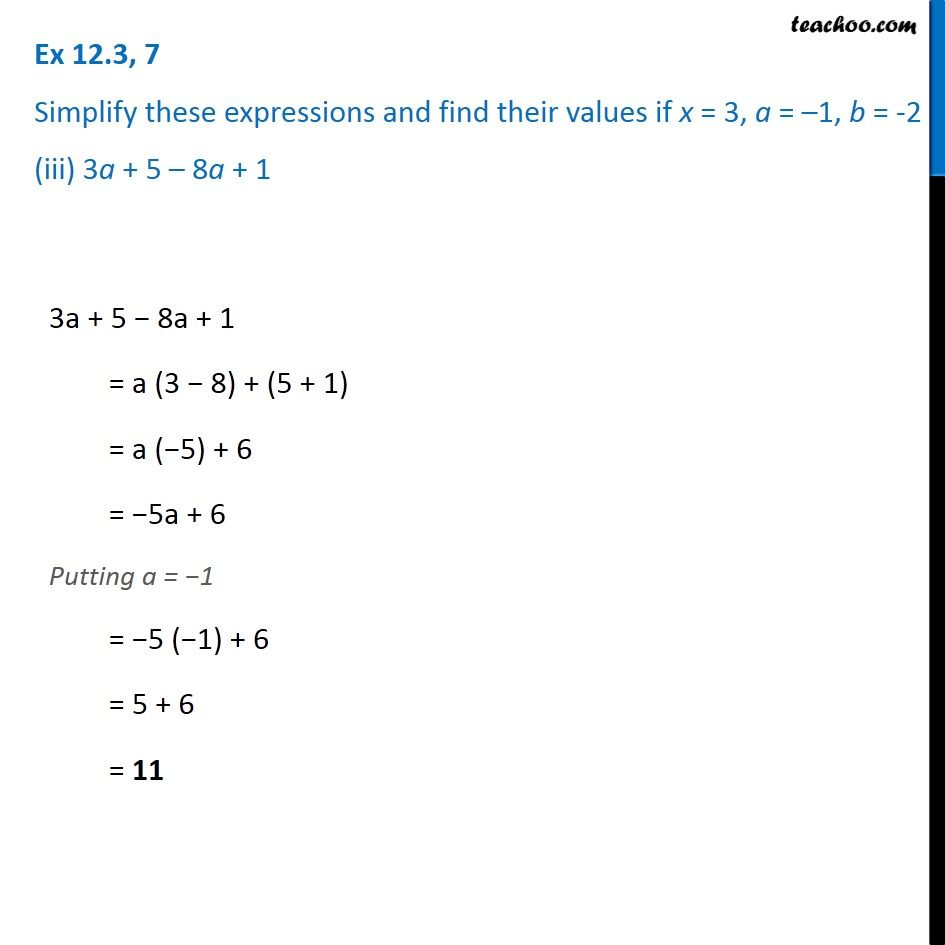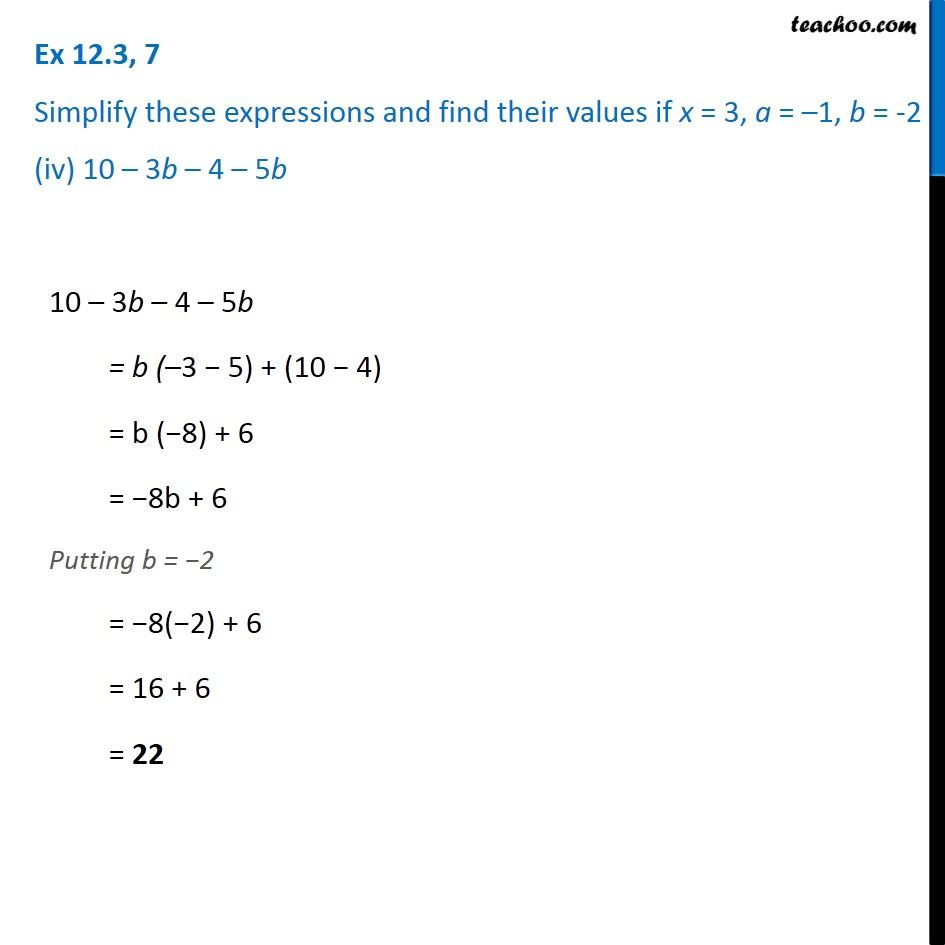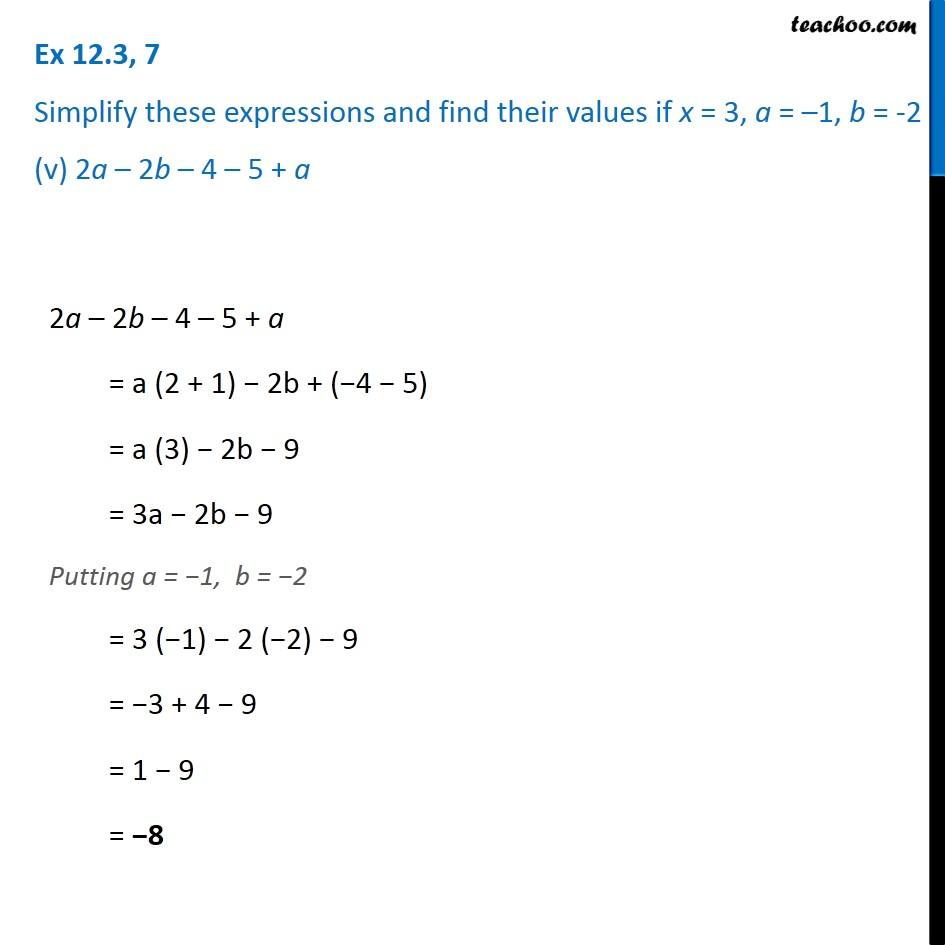Subscribe to our Youtube Channel - https://you.tube/teachoo

1. Chapter 12 Class 7 Algebraic Expressions
2. Serial order wise
3. Ex 12.3

Transcript

Ex 12.3, 7 Simplify these expressions and find their values if x = 3, a = –1, b = -2 (i) 3x – 5 – x + 9 3x – 5 – x + 9 = x (3 – 1) + (–5 + 9) = x (2) + 4 = 2x + 4 Putting 𝑥 = 3 = 2 (3) + 4 = 6 + 4 = 10 Ex 12.3, 7 Simplify these expressions and find their values if x = 3, a = –1, b = -2 (ii) 2 – 8x + 4x + 4 2 − 8x + 4x + 4 = x (−8 + 4) + (4 + 2) = x (−4) + 6 = −4x + 6 Putting 𝑥 = 3 = −4(3) + 6 = −12 + 6 = −6 Ex 12.3, 7 Simplify these expressions and find their values if x = 3, a = –1, b = -2 (iii) 3a + 5 – 8a + 1 3a + 5 − 8a + 1 = a (3 − 8) + (5 + 1) = a (−5) + 6 = −5a + 6 Putting a = −1 = −5 (−1) + 6 = 5 + 6 = 11 Ex 12.3, 7 Simplify these expressions and find their values if x = 3, a = –1, b = -2 (iv) 10 – 3b – 4 – 5b 10 – 3b – 4 – 5b = b (–3 − 5) + (10 − 4) = b (−8) + 6 = −8b + 6 Putting b = −2 = −8(−2) + 6 = 16 + 6 = 22 Ex 12.3, 7 Simplify these expressions and find their values if x = 3, a = –1, b = -2 (v) 2a – 2b – 4 – 5 + a 2a – 2b – 4 – 5 + a = a (2 + 1) − 2b + (−4 − 5) = a (3) − 2b − 9 = 3a − 2b − 9 Putting a = −1, b = −2 = 3 (−1) − 2 (−2) − 9 = −3 + 4 − 9 = 1 − 9 = −8

Ex 12.3